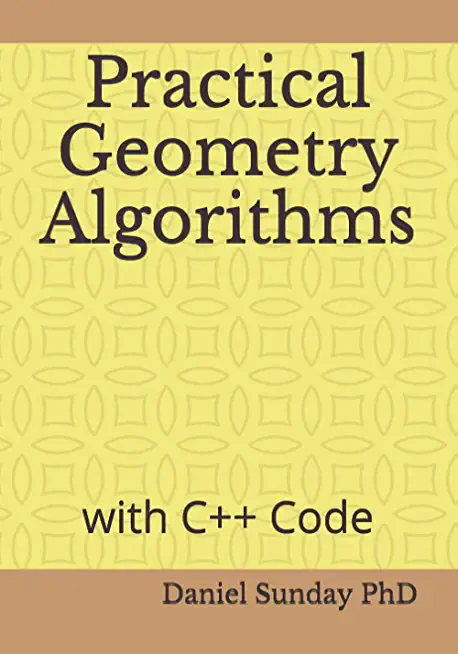### Practical Geometry Algorithms: with C++ Code### Huge savings for students

Each student receives a 50% discount off of most books in the HSG Book Store. During class, please ask the instructor about purchase details.
 List Price: \$16.95 Price: \$8.48 You Save: \$8.48
5This book presents practical geometry algorithms with computationally fast C++ code implementations. It covers algorithms for fundamental geometric objects, such as points, lines, rays, segments, triangles, polygons, and planes. These algorithms determine the basic 2D and 3D properties, such as area, distance, inclusion, and intersections. There are also algorithms to compute bounding containers for these objects, including a fast bounding ball, various convex hull algorithms, as well as polygon extreme points and tangents. And there is a fast algorithm for polyline simplification using decimation that works in any dimension.

These algorithms have been used in practice for several decades. They are robust, easy to understand, code, and maintain. And they execute very rapidly in practice, not just in theory. For example, the winding number point in polygon inclusion test, first developed by the author in 2000, is the fastest inclusion algorithm known, and works correctly even for non-simple polygons. Also, there is a fast implementation of the Melkman algorithm for the convex hull of a simple polyline. And much more.

If your programming involves geometry, this book will be an invaluable reference. Further, along with the book, there is a free download of all the C++ code from the book, plus some additional supporting code.
Independently Published